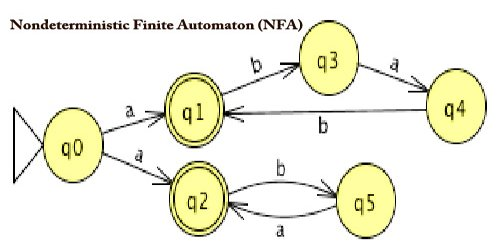Computer

# Nondeterministic Finite Automaton (NFA)Nondeterministic finite automaton (NFA) or nondeterministic finite-state machine (NFSM) is a model of computation, and it does not need to obey these restrictions. In particular, every DFA (deterministic finite automaton) is additionally an NFA. In an exceedingly nondeterministic finite automaton (NFA), for every state, there are often zero, one, two, or more transitions corresponding to a particular symbol. NFAs are often constructed from any regular expression using Thompson’s construction algorithm.

Nondeterministic Finite Automata (NFA) is defined by the quintuple –

M = (Q, ∑, δ, q0, F)

Where –

Q = finite set of states

∑ = non-empty finite set of symbols called as input alphabets

δ : Q x ∑ → 2Q is a total function called a transition function

q0 ∈ Q is the initial state

F ⊆ Q is a set of final states

A Non-deterministic Finite Automaton (NFA) is represented by digraphs called state diagram.

• The vertices represent the states.
• The arcs labeled with an input alphabet show the transitions.
• The initial state is denoted by an empty single incoming arc.
• The final state is indicated by double circles.

NFAs was introduced in 1959 by Michael O. Rabin and Dana Scott, who also showed their equivalence to DFAs. NFAs are used in the implementation of regular expressions: Thompson’s construction is an algorithm for compiling a regular expression to an NFA that can efficiently perform pattern matching on strings. Conversely, Kleene’s algorithm can be used to convert an NFA into a regular expression (whose size is generally exponential in the input automaton).

Different views of non-determinism finite automaton (NFA) –

• The NFA always makes the right choice (of a successor state according to δ) to insure reaching the final state (if possible at all)
• The NFA simultaneously explores multiple paths
• At each nondeterministic choice point, the NFA spawns off multiple copies of itself

The various path/computations evolve completely independently from each other (this is different from parallel computations which may synchronize at a certain point). NFAs are generalized in multiple ways, e.g., a nondeterministic finite automaton with ε-moves, finite-state transducers, pushdown automata, alternating automata, ω-automata, and probabilistic automata. Besides the DFAs, other known special cases of NFAs are unambiguous finite automata (UFA) and self-verifying finite automata (SVFA).

Nondeterministic finite automaton with ε-moves (NFA-ε) is a further generalization to NFA. This automaton replaces the transition function with the one that allows the empty string ε as a possible input. The transitions without consuming an input symbol are called ε-transitions. In the state diagrams, they’re usually labeled with the Greek letter ε. ε-transitions provide a convenient way of modeling the systems whose current states don’t seem to be precisely known: i.e., if we are modeling a system and it’s not clear whether the present state (after processing some input string) should be q or q’, then we are able to add an ε-transition between these two states, thus putting the automaton in both states simultaneously.

Nondeterministic finite automaton (NFAs) and deterministic finite automaton (DFAs) are equivalent in that if a language is recognized by an NFA, it’s also recognized by a DFA and the other way around. The establishment of such equivalence is very important and useful. It’s useful because constructing an NFA to acknowledge a given language is typically much easier than constructing a DFA for that language. It’s important because NFAs may be wont to reduce the complexity of the mathematical work required to determine many important properties within the theory of computation. For instance, it’s much easier to prove the closure properties of standard languages using NFAs than DFAs.

Information Sources: